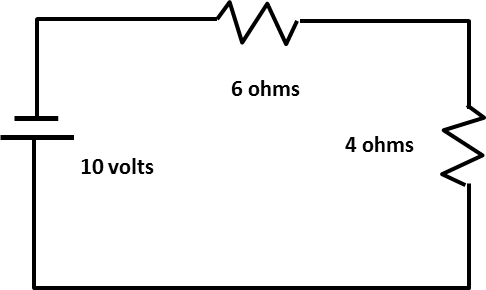The current in the 4 ohm resistor is ______.
Use Ohm's law : V=IR. The total resistance in the circuit is 10 ohms (add the resistance for serial resistors). Therefore the total current across either of the resistors is $$I = \frac{\mathrm{V} }{\mathrm{R} } = \frac{\mathrm{10} }{\mathrm{10} } = 1$$ amp.